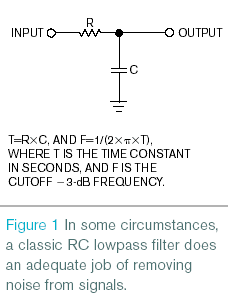# Simple Digital Filter Cleans Up Noisy Data

Many systems use an ADC to sample analog data that temperature and pressure sensors produce. Sometimes, system noise or other factors cause the otherwise slowly fluctuating data to "jump around". To reduce higher frequency noise, designers often install an analog RC (resistor-capacitor) lowpass filter between the sensor and the analog-to-digital-conversion stage. However, this approach is not always ideal or practical. For example, a long time constant of minutes would require very large values for R and C.

Figure 1 shows an analog RC lowpass filter and its design equations. As an alternative, you can clean up noisy signals that remain within the ADC's linear range by using the digital equivalent of an analog RC lowpass filter. The filter's software comprises only two lines of C code: LPOUT=LPACC/K, where the output value of the filter is LPACC divided by a constant, and LPACC=LPACC+LPIN–LPOUT, where you add the difference between input and output to update LPACC. You specify all variables as integers.Each time the analog-to-digital conversion acquires a new input sample, LPIN, the software produces an output value, LPOUT, which comprises a lowpass-filtered version of the input samples. Calculate the value of the constant, K, based on the sampling rate of the system and the desired time constant for the filter as follows: K=T × SPS, where K>1, and SPS is the system's sampling rate. For example, for a system-sampling rate of 200 samples/sec and a desired time constant of 30 sec, the constant K would equal 6000 samples. Applying a step change to the routine's input requires 6000 samples to reach approximately 63 % of its final value at the output.

The lowpass accumulator, LPACC, can grow large for large time constants and large input values. It can grow as large as K times the largest possible LPIN value. Under these conditions, you need to make sure that LPACC does not overflow, and you may need to specify a larger data type to contain LPACC. To avoid a long settling time during start-up, before the start of the sampling loop, you can initialize LPACC to a value of K times the current input value.

You can extend the basic filter concept presented to accommodate higher order filters with greater high-frequency rejection by executing multiple filter code segments in sequence. Also, you can use an array of variables for LPACC and an array of values of the constant K to filter signals that multiple data channels acquire.

EDN